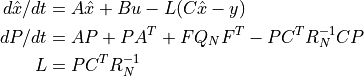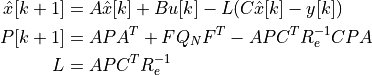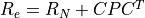# control.create_estimator_iosystem¶

control.create_estimator_iosystem(sys, QN, RN, P0=None, G=None, C=None, control_indices=None, disturbance_indices=None, estimate_labels='xhat[{i}]', covariance_labels='P[{i},{j}]', measurement_labels=None, control_labels=None, inputs=None, outputs=None, states=None, **kwargs)[source]

Create an I/O system implementing a linear quadratic estimator

This function creates an input/output system that implements a continuous time state estimator of the formor a discrete time state estimator of the formwhere. It can be called in the form:

estim = ct.create_estimator_iosystem(sys, QN, RN)


where sys is the process dynamics and QN and RN are the covariance of the disturbance noise and measurement noise. The function returns the estimator estim as I/O system with a parameter correct that can be used to turn off the correction term in the estimation (for forward predictions).

Parameters
• sys (LinearIOSystem) – The linear I/O system that represents the process dynamics.

• QN (ndarray) – Disturbance and measurement noise covariance matrices.

• RN (ndarray) – Disturbance and measurement noise covariance matrices.

• P0 (ndarray, optional) – Initial covariance matrix. If not specified, defaults to the steady state covariance.

• G (ndarray, optional) – Disturbance matrix describing how the disturbances enters the dynamics. Defaults to sys.B.

• C (ndarray, optional) – If the system has full state output, define the measured values to be used by the estimator. Otherwise, use the system output as the measured values.

• control_indices (int, slice, or list of int or string, optional) – Specify the indices in the system input vector that correspond to the control inputs. These inputs will be used as known control inputs for the estimator. If value is an integer m, the first m system inputs are used. Otherwise, the value should be a slice or a list of indices. The list of indices can be specified as either integer offsets or as system input signal names. If not specified, defaults to the system inputs.

• disturbance_indices (int, list of int, or slice, optional) – Specify the indices in the system input vector that correspond to the unknown disturbances. These inputs are assumed to be white noise with noise intensity QN. If value is an integer m, the last m system inputs are used. Otherwise, the value should be a slice or a list of indices. The list of indices can be specified as either integer offsets or as system input signal names. If not specified, the disturbances are assumed to be added to the system inputs.

• estimate_labels (str or list of str, optional) – Set the names of the state estimate variables (estimator outputs). If a single string is specified, it should be a format string using the variable i as an index. Otherwise, a list of strings matching the number of system states should be used. Default is “xhat[{i}]”.

• covariance_labels (str or list of str, optional) – Set the name of the the covariance state variables. If a single string is specified, it should be a format string using the variables i and j as indices. Otherwise, a list of strings matching the size of the covariance matrix should be used. Default is “P[{i},{j}]”.

• measurement_labels (str or list of str, optional) – Set the name of the measurement and control signal names (estimator inputs). If a single string is specified, it should be a format string using the variable i as an index. Otherwise, a list of strings matching the size of the system inputs and outputs should be used. Default is the signal names for the system measurements and known control inputs. These settings can also be overriden using the inputs keyword.

• control_labels (str or list of str, optional) – Set the name of the measurement and control signal names (estimator inputs). If a single string is specified, it should be a format string using the variable i as an index. Otherwise, a list of strings matching the size of the system inputs and outputs should be used. Default is the signal names for the system measurements and known control inputs. These settings can also be overriden using the inputs keyword.

• inputs (int or list of str, optional) – Set the names of the inputs, outputs, and states, as described in InputOutputSystem(). Overrides signal labels.

• outputs (int or list of str, optional) – Set the names of the inputs, outputs, and states, as described in InputOutputSystem(). Overrides signal labels.

• states (int or list of str, optional) – Set the names of the inputs, outputs, and states, as described in InputOutputSystem(). Overrides signal labels.

• name (string, optional) – System name (used for specifying signals). If unspecified, a generic name <sys[id]> is generated with a unique integer id.

Returns

estim – Input/output system representing the estimator. This system takes the system output y and input u and generates the estimated state xhat.

Return type

InputOutputSystem

Notes

This function can be used with the create_statefbk_iosystem() function to create a closed loop, output-feedback, state space controller:

K, _, _ = ct.lqr(sys, Q, R)
est = ct.create_estimator_iosystem(sys, QN, RN, P0)
ctrl, clsys = ct.create_statefbk_iosystem(sys, K, estimator=est)


The estimator can also be run on its own to process a noisy signal:

resp = ct.input_output_response(est, T, [Y, U], [X0, P0])


If desired, the correct parameter can be set to False to allow prediction with no additional measurement information:

resp = ct.input_output_response(
est, T, 0, [X0, P0], param={'correct': False)